# High School Physics : Interpreting Force Diagrams

## Example Questions

### Example Question #11 : Net Force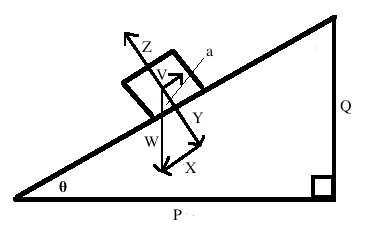Which of the following represents the force due to friction?

P

Z

V

Y

X

V

Explanation:

The force due to friction will always be parallel to the surface upon which the object is traveling. It will come directly from the center of the object, and be pointed in the opposite direction to the motion of the object.

In this diagram, V is pointed parallel to the surface and opposite to the direction of motion.

### Example Question #11 : Net ForceWhich of the following represents the force due to gravity?

W

X

Z

Y

V

W

Explanation:

Remember, in a force diagram, forces come from the center of the object. The force of gravity will always be straight down from the center of the object.

In this diagram, W is pointed directly downward.

### Example Question #13 : Net ForceWhat is the value of anglewithin the triangle made by X, W, and Y?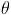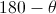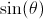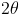Explanation:

When working in an inclined plane, when we break our force due to gravity into components, the angle at the top that triangle will be equal to the angle of the inclined plane.

In the diagram, the total force due to gravity is given by WX represents the horizontal component, and is parallel to the surface of the plane. Y represents the vertical component, and is perpendicular to the surface of the plane. X and Y thus create a right angle, with W as the hypotenuse. By turning this triangle such that the right angle aligns with the right angle of the inclined plane (between Q and P), we can see that W aligns with the incline surface and Y aligns with the base, P. Based on these alignments, the angle between W and Y must be equal to the angle between the surface and P; thus,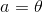.

### Example Question #14 : Net ForceWhich of the following represents the normal force?

X

Z

-W

Q

V

Z

Explanation:

The normal force is always perpendicular to the surface on which the object is placed, and is pointed away from said surface.

In this diagram, Z is the only force that is perpendicular to the surface and in the upward direction. This force must counteract the vertical force of gravity, which will be perpendicular to the surface in the downward direction (Y).

### Example Question #101 : ForcesWhich of the following statements is not true?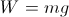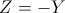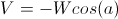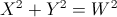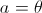Explanation:

In this diagram, V represents the force due to friction. The equation for the force due to friction is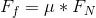, where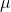is the coefficient of friction.

In this case, Z represents the normal force. We can re-write the equation for friction: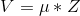Z can be re-written in terms of the angle, but will always need to be multiplied by the coefficient of friction in order to give an equation for V.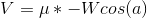The other equations are true.- X and Y form a right angle, so the Pythagorean theorem applies.- Z is the normal force, which is, by definition, equal and opposite the vertical force of gravity.- W is the total force of gravity, which will be equal to the mass times the acceleration of gravity.- the triangle formed by W, X, and Y is similar to the triangle formed by the surface, Q, and P, meaning that these angles must be equal.

### Example Question #22 : Net ForceAccording to this force diagram, the normal force will be equal to which of the following?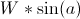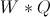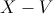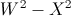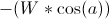Explanation:

In this problem, Y and Z are equal, but opposite forces, and Z is our normal force.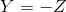If we can solve for Y, then we can find Z.

We can use our understanding of trigonometry to find an equation for Y.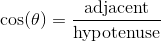If we plug infor the angle, we see: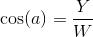Since we are solving for Y, we can multiply both sides by W.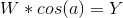Now that we know an equation for Y, we can return to our original equation to solve for Z.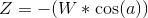### Example Question #23 : Net ForceWhat is the distance the object travels based on this diagram?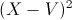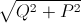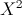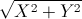Explanation:

There is no way for us to know the distance the object travels. Even though the force diagram places our object roughly halfway up the plane, we do not know the position where the object starts or where the object ends. We cannot calculate a change in distance.

### Example Question #24 : Net ForceWhich of the following correctly calculates X in terms of W?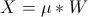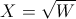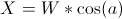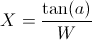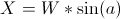Explanation:

We can use our understanding of trigonometry to find this relationship, since X and Y form a right angle.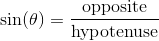If we plug infor the angle, we see that X is opposite ofand W is the hypotenuse.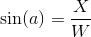Since we're looking for X, we can multiply both sides by W.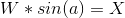### Example Question #25 : Net ForceHow can we use this diagram to help calculate the net force on the object?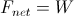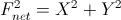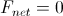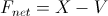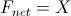Explanation:

For this problem, we need to break the forces into horizontal and vertical components. The only difference with an inclined plane is that you have to translate the horizontal plane to be parallel to the surface upon which the object is traveling, and the vertical plane to be perpendicular to the surface upon which the object is traveling.

Luckily, this is already done in the diagram, with the force of gravity (W) broken into the vertical component (Y) and horizontal component (X).

Now, we need to sum the forces in each plane. The forces in the vertical plane will be perpendicular to the surface: Z and Y.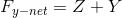Since Z and Y are opposite and equal forces: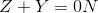There is no net force in the vertical plane. This makes sense, as we would not expect the object to have any movement perpendicular to the surface on which it is sliding.

Now we can sum the horizontal forces: V and X.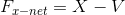Note that X is positive and V is negative. This is because V represents the force of friction, and is opposite to the force of X.

Since there is no force in the vertical plane, this gives our final net force: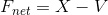.